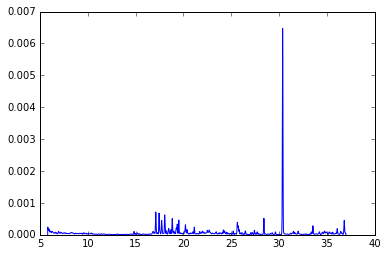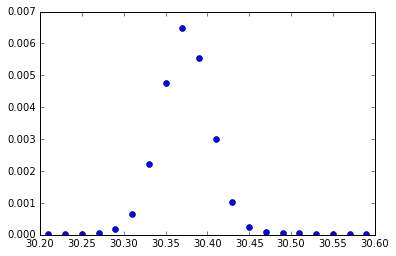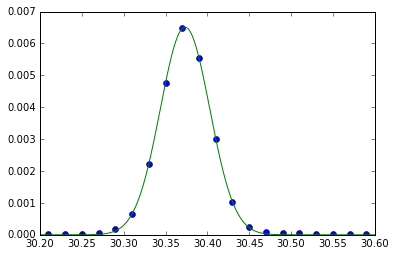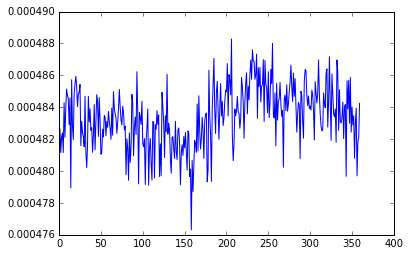In :
%matplotlib inline


# Solar Data Processing with Python Part II¶

Now we have a grasp of the basics of python, but the whole reason for downloading python in the first place was to analyze solar data. Let's take a closer look at examples of solar data analysis.

We will be using SunPy to access solar data. SunPy is a python package designed to interface between the powerful tools that exist in other Python Libraries with current repositories of solar data. With SunPy we will show how to: download solar data sets from the VSO, calibrate to industry standards, plot and overlay a time series.

# Fitting A Gaussian to a Spectral Line¶

One of the most common data types in solar data processing is a time series. A time series is a measurement of how one physical parameter changes as a function of time. This example shows how to fit a gaussian to a spectral line. In this example, it will be as "real world" as possible.

First, let's import some useful libraries.

In :
from datetime import datetime, timedelta #we saw these in the last tutorial

import numpy as np
from astropy.io import fits #how to read .fits files
from astropy.modeling import models, fitting #some handy fitting tools from astropy
import matplotlib.pyplot as plt
from scipy.integrate import trapz #numerical itegration tool
import astropy.units as u #units!!


Next we need to load in the data set we want to work with:

In :
filename = '/Users/mskirk/Documents/Conferences/SDO 2016/EVS_L2_2011045_01_005_01.fits'

In :
hdulist = fits.open(filename)


So what did we get when we opened the file? Let's take a look:

In :
len(hdulist)

Out:
4

We got 4 items in the list. Lets take a look at the first one:

In :
hdulist.header

Out:
SIMPLE  =                    T /Dummy Created by MWRFITS v1.11
BITPIX  =                    8 /Dummy primary header created by MWRFITS
NAXIS   =                    0 /No data is associated with this header
EXTEND  =                    T /Extensions may (will!) be present               

This doesn't contain any useful information. Let's take a look at the second item:

In :
hdulist.header

Out:
XTENSION= 'BINTABLE'           /Binary table written by MWRFITS v1.11
BITPIX  =                    8 /Required value
NAXIS   =                    2 /Required value
NAXIS1  =                    8 /Number of bytes per row
NAXIS2  =                 5200 /Number of rows
PCOUNT  =                    0 /Normally 0 (no varying arrays)
GCOUNT  =                    1 /Required value
TFIELDS =                    2 /Number of columns in table
COMMENT
COMMENT  *** End of mandatory fields ***
COMMENT
EXTNAME = 'SpectrumMeta'
TUNIT1  = 'nm'
COMMENT
COMMENT  *** Column names ***
COMMENT
TTYPE1  = 'WAVELENGTH'         /
TTYPE2  = 'ACCURACY'           /
COMMENT
COMMENT  *** Column formats ***
COMMENT
TFORM1  = 'E       '           /
TFORM2  = 'E       '           /
COMMENT  Wavelength (nm) is the center value of each bin in the Spectrum table
COMMENT  Accuracy is the relative accuracy of the entire MEGS measurement at
COMMENT    each wavelength.                                                     

Alright, now we are getting somewhere. This has wavelength information in units of 'nm' and accuracy information without units. Let's take a look at the other elements of the list we got:

In :
hdulist.header

Out:
XTENSION= 'BINTABLE'           /Binary table written by MWRFITS v1.11
BITPIX  =                    8 /Required value
NAXIS   =                    2 /Required value
NAXIS1  =                  786 /Number of bytes per row
NAXIS2  =                    1 /Number of rows
PCOUNT  =                    0 /Normally 0 (no varying arrays)
GCOUNT  =                    1 /Required value
TFIELDS =                   10 /Number of columns in table
COMMENT
COMMENT  *** End of mandatory fields ***
COMMENT
EXTNAME = 'SpectrumUnits'
COMMENT
COMMENT  *** Column names ***
COMMENT
TTYPE1  = 'TAI     '           /
TTYPE2  = 'YYYYDOY '           /
TTYPE3  = 'SOD     '           /
TTYPE4  = 'FLAGS   '           /
TTYPE5  = 'SC_FLAGS'           /
TTYPE6  = 'INT_TIME'           /
TTYPE8  = 'COUNT_RATE'         /
TTYPE9  = 'PRECISION'          /
TTYPE10 = 'BIN_FLAGS'          /
COMMENT
COMMENT  *** Column formats ***
COMMENT
TFORM1  = '87A     '           /
TFORM2  = '68A     '           /
TFORM3  = '65A     '           /
TFORM4  = '55A     '           /
TFORM5  = '87A     '           /
TFORM6  = '39A     '           /
TFORM7  = '149A    '           /
TFORM8  = '61A     '           /
TFORM9  = '122A    '           /
TFORM10 = '53A     '           /
COMMENT  First element is unit
COMMENT  Other elements are brief descriptions and other info                   
In :
hdulist.header

Out:
XTENSION= 'BINTABLE'           /Binary table written by MWRFITS v1.11
BITPIX  =                    8 /Required value
NAXIS   =                    2 /Required value
NAXIS1  =                67630 /Number of bytes per row
NAXIS2  =                  360 /Number of rows
PCOUNT  =                    0 /Normally 0 (no varying arrays)
GCOUNT  =                    1 /Required value
TFIELDS =                   10 /Number of columns in table
COMMENT
COMMENT  *** End of mandatory fields ***
COMMENT
ORIGIN  = '            SDO/EVE SPOC' // LASP, University of Colorado, Boulder
DATE    = '2014-12-03T15:32:19.000Z' // UTC file creation time (per ISO8601)
TAI_OBS =            1676336446.501 // TAI time at start of obs
DATE_OBS= '2011-02-14T01:00:12.501Z' // UTC at start of obs
T_OBS   = '2011-02-14T01:00:17.501Z' // UTC at center of obs
EXPTIME =                    10.000 // seconds exposed, or integration time
TIME    =                  3612.501 // UTC seconds of day at start of obs
TELESCOP= '            SDO/EVE'
INSTRUME= '        EVE_MEGS'
VERSION =                        05 // major code/cal version
REVISION=                         1 // reprocess number
FILENAME= 'EVS_L2_2011045_01_005_01.fit'
COMMENT
COMMENT  *** Column names ***
COMMENT
TTYPE1  = 'TAI     '           /
TTYPE2  = 'YYYYDOY '           /
TTYPE3  = 'SOD     '           /
TTYPE4  = 'FLAGS   '           /
TTYPE5  = 'SC_FLAGS'           /
TTYPE6  = 'INT_TIME'           /
TTYPE8  = 'COUNT_RATE'         /
TTYPE9  = 'PRECISION'          /
TTYPE10 = 'BIN_FLAGS'          /
COMMENT
COMMENT  *** Column formats ***
COMMENT
TFORM1  = 'D       '           /
TFORM2  = 'J       '           /
TFORM3  = 'D       '           /
TFORM4  = 'B       '           /
TFORM5  = 'B       '           /
TFORM6  = 'D       '           /
TFORM7  = '5200E   '           /
TFORM8  = '5200E   '           /
TFORM9  = '5200E   '           /
TFORM10 = '5200B   '           /
COMMENT EVE Principal Investigator T. N. Woods
COMMENT Laboratory for Atmospheric and Space Physics
COMMENT 1234 Innovation Drive, Boulder, CO 80303
COMMENT SDO Mission scientific and model results are open to all.
COMMENT Users should contact the PI or designated EVE team member early in an
COMMENT analysis project to discuss appropriate use of instrument data results.
COMMENT Appropriate acknowledgement to institutions, personnel, and funding
COMMENT agencies should be given. Version numbers should also be specified.
COMMENT Pre-prints of publications and conference abstracts should be widely
COMMENT distributed to interested parties within the mission.
EXTNAME = 'Spectrum'
COMMENT  Wavelength scale is as described in SpectrumMeta table
COMMENT  Count_Rate is the dark corrected counts per second per pixel
COMMENT    for pixels determined to be within the wavelength bin.
COMMENT  Data from short wavelengths up to and including 17.24 nm
COMMENT    are from MEGS-A slit 1. Data from above that point to 37.00 nm
COMMENT    are from MEGS-A slit 2. Data above that point are from MEGS-B.
COMMENT  MEGS-B has reduced exposure time to increase detector life.
COMMENT  Values in FLAGS field are the bitwise OR of these values.
COMMENT  Bit 0 (value    1) - MEGS-A data is missing
COMMENT  Bit 1 (value    2) - MEGS-B data is missing
COMMENT  Values in SC_FLAGS field are the bitwise OR of these values.
COMMENT  Bit 0 (value    1) - 4-bit obstruction indicator (0 is no obstruction)
COMMENT  Bit 1 (value    2) - 4-bit obstruction indicator (0 is no obstruction)
COMMENT  Bit 2 (value    4) - 4-bit obstruction indicator (0 is no obstruction)
COMMENT  Bit 3 (value    8) - 4-bit obstruction indicator (0 is no obstruction)
COMMENT  Bit 4 (value   16) - Observatory is off-pointed by more than 1 arcmin
COMMENT  Obstruction flag values:
COMMENT  Value 0 No obstruction
COMMENT  Value 1 Warmup from Earth eclipse
COMMENT  Value 2 Atmosphere penumbra
COMMENT  Value 3 Atmosphere umbra
COMMENT  Value 4 Penumbra of Mercury
COMMENT  Value 5 Umbra of Mercury
COMMENT  Value 6 Penumbra of Venus
COMMENT  Value 7 Umbra of Venus
COMMENT  Value 8 Penumbra of Moon
COMMENT  Value 9 Umbra of Moon
COMMENT  Value 10 Penumbra of solid Earth
COMMENT  Value 11 Umbra of solid Earth
COMMENT  If more than one obstruction is taking place, only
COMMENT  the highest-numbered one will be indicated.                            

So it looks like we are working with some wavelength data, spectral information, irradiance data, etc.

# Plotting Spectral Data¶

Let's take a look at some of the data we've got.

In :
len(hdulist.data)

Out:
5200
In :
hdulist.data["WAVELENGTH"]

Out:
array([   3.00999999,    3.02999997,    3.04999995, ...,  106.94999695,
106.97000122,  106.98999786], dtype=float32)
In :
len(hdulist.data)

Out:
360
In :
hdulist.data["IRRADIANCE"]

Out:
array([-1., -1., -1., ..., -1., -1., -1.], dtype=float32)
In :
plt.plot(hdulist.data["WAVELENGTH"], hdulist.data["IRRADIANCE"])
plt.ylim(0, 0.007)
plt.xlim(5, 40)

Out:
(5, 40)So now we have a plot of wavelength vs. irradiance. We can see there is one major spike in the data. Let's filter the data so that we just have that one spike.

In :
w = np.logical_and(hdulist.data["WAVELENGTH"] > 30.2, hdulist.data["WAVELENGTH"] < 30.6)

In :
w

Out:
array([False, False, False, ..., False, False, False], dtype=bool)

This function, "np.logical_and", is similar to a "where" statement in IDL. We can see that "w" is now an array of true and false values. To take a subsection of our data where our filter is true:

In :
wavelength = hdulist.data["WAVELENGTH"][w]

In :
wavelength

Out:
array([ 30.20999908,  30.22999954,  30.25      ,  30.27000046,
30.29000092,  30.30999947,  30.32999992,  30.35000038,
30.37000084,  30.38999939,  30.40999985,  30.43000031,
30.45000076,  30.46999931,  30.48999977,  30.51000023,
30.53000069,  30.54999924,  30.56999969,  30.59000015], dtype=float32)

Now, we need to add some units to this data. The header of the file tells us what the units are:

In :
wavelength = u.Quantity(wavelength, unit="nm")


Let's do the same thing to the irradiance data.

In :
irr = hdulist.data["IRRADIANCE"][w]

In :
irr = u.Quantity(irr, unit="W/m**2")


What have we got? Let's plot it and take a look:

In :
plt.plot(wavelength, irr, 'o')

Out:
[<matplotlib.lines.Line2D at 0x120092350>]# Fit He II 304 line with a Gaussian¶

Now that we have extracted the He II line from our total spectrum, we want to fit it with a gaussian. Do do this we will make use of a couple of packages in in astropy. We will initialize the gaussian fit with some approximations (max, center, FWHM):

In :
g_init = models.Gaussian1D(0.007, 30.4, .2)


Now let's define a fitting method and produce a fit:

In :
fit_g = fitting.LevMarLSQFitter()

In :
g = fit_g(g_init, wavelength, irr)


Let's take a look at some of the qualities of our fitted gaussian:

In :
g.mean

Out:
Parameter('mean', value=30.3733491452)
In :
g.stddev

Out:
Parameter('stddev', value=0.029547785343)
In :
g.amplitude

Out:
Parameter('amplitude', value=0.00651223358276)
In :
g

Out:
<Gaussian1D(amplitude=0.006512233582758824, mean=30.373349145178118, stddev=0.029547785343013666)>

Our guesses wern't too bad, but we over estimated the Standard Deviation by about a factor of 10. The variable 'g' has the fitted parameters of our gaussian but it doesn't actually contain an array. To plot it over the data, we need to create an array of values. We will make an array from 30.2 to 30.6 with 1000 points in it.

In :
x = np.linspace(30.2, 30.6, 1000)


To find the values of our fit at each location, it is easy:

In :
y = g(x)


Now we can plot it:

In :
plt.plot(wavelength, irr, 'o')
plt.plot(x, y)

Out:
[<matplotlib.lines.Line2D at 0x12085b090>]# Ingegrating under the curve.¶

Let's find the area under the curve we just created. We can numerically integrate it easily:

In :
intensity = trapz(y,x)

In :
intensity

Out:
0.00048233062533621857

# Produce a Light Curve of He II 304 From All Spectra in File¶

The file we downloaded is a hyper-spectrum. This means that the spectrum changes over time. Can we find how the intensity of the line changes over time using the same fitting tools that we just showcased? Sure, we just need to put everything into a loop.

In :
x = np.linspace(30.2, 30.6, 1000) #define our wavelength array
intensity = [] # initialize the intensity array
w = np.logical_and(hdulist.data["WAVELENGTH"] > 30.2, hdulist.data["WAVELENGTH"] < 30.6) # isolate the 304 line
wavelength = hdulist.data["WAVELENGTH"][w] # filter all of the spectra
fit_g = fitting.LevMarLSQFitter() #fitting algorithm

for spectrum in hdulist.data: #looping over all the spectra (360)
g_init = models.Gaussian1D(0.007, 30.4, .2) #initialize the gaussian fit
g = fit_g(g_init, wavelength, irr) #do the fitting
y = g(x) # create the curve
intensity.append(trapz(y,x)) # aggregate the results
intensity = u.Quantity(intensity, unit="nm") # adding units.

In :
plt.plot(intensity)

Out:
[<matplotlib.lines.Line2D at 0x1222f4ed0>]In [ ]: